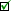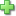Life of Fred: Beginning Algebra

(as serious as it needs to be)

Life of Fred
by Stanley F. Schmidt
Hardcover, 544 pages
Price: \$39.00
Used Price: \$25.00 (1 in stock)Condition Policy

Please do not start Life of Fred: Beginning Algebra without doing the two pre-algebra books first (or their equivalent). The two pre-algebra books are an essential introduction to the material of beginning algebra. They teach, among many other things,

• Graphing
• An introduction to word problems.

For example, one of the problems in a Your Turn to Play is:

Let's suppose on some day Fred sold x Gourmet Gauss Dogs and made a profit of \$3 per dog. On that day he also sold x- 2 Double Dogs and made a profit of \$2 per dog. And on that day he sold x - 8 Cold Dogs and made a profit of \$1 per dog. If he made \$168 on that day, how many Gourmet Gauss Dogs did he sell?

The Fred booksare serious about learning how to do word problems.

Life of Fred: Beginning Algebra offers more material than is normally taught in a year of high school algebra. It also chronicles five days of Fred's life when he is drafted by accident (at the age of 6) and sent off to an army camp in Texas.

Chapter 1 - Numbers and Sets

finite and infinite sets
natural numbers, whole numbers, integers
set notation
negative numbers
ratios
the empty set

Chapter 2 - The Integers

less than (<) and the number line
multiplication
proportion
π
coefficients

Chapter 3 - Equations

solving equations with ratios
formulas from geometry
order of operations
consecutive numbers
rational numbers
set builder notation
distance = (rate)(time) problems
distributive property
proof that (negative) × (negative) = positive

Chapter 4 - Motion and Mixture

proof of the distributive property
price and quantity problems
mixture problems
age problems

Chapter 5 - Two Unknowns

solving two equations, two unknowns by elimination
union of sets
graphing of points
mean, mode, and median averages
graphing linear equations
graphing any equation

Chapter 6 - Exponents

solving two equations, two unknowns by graphing
solving two equations, two unknowns by substitution
xmxn, (xm)n and xm ÷ xn
inconsistent and dependent equations
factorials
commutative laws
negative exponents

Chapter 7 - Factoring

multiplying binomials
common factors
factoring x² + bx + c
factoring a difference of squares
factoring by grouping
factoring ax² + bx + c

Chapter 8 - Fractions

solving equations containing fractions
simplifying fractions
multiplying and dividing fractions
complex fractions

Chapter 9 - Square Roots

principal square roots
Pythagorean theorem
the real numbers
the irrational numbers
cube roots and indexes
rationalizing the denominator
extraneous roots

solving quadratic equations by completing the square
long division of a polynomial by a binomial

Chapter 11 - Functions and Slope

definition of a function
domain, codomain, image
six definitions of slope
slope-intercept (y = mx + b) form of the line
range of a function

Chapter 12 - Inequalities and Absolute Value

graphing inequalities in two dimensions
division by zero
algebraically solving linear inequalities with one unknown

A.R.T. section (quick summary of all of beginning algebra)

Index

• Numbers
• Integers
• Equations
• Motion & Mixture
• Two Unknowns
• Exponents
• Factoring
• Fractions
• Square RootsClick here to write a review